# How to Calculate and Solve for Maximum Velocity, Coefficient of Friction and Radius of a Body in Motion of Circular Path | Nickzom Calculator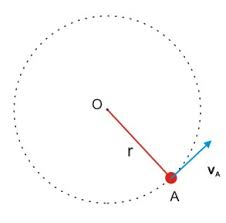The image above represents maximum velocity in circular motion.

To compute for the maximum velocity, three essential parameters are needed and these parameters are coefficient of friction (μ), radius (r) and acceleration due to gravity (g).

The formula for calculating maximum velocity:

Vmax = √(μgr)

Where;
Vmax = maximum velocity
μ = coefficient of friction
g = acceleration due to gravity

Let’s solve an example;
Find the maximum velocity when the coefficient of friction is 14 with a radius of 7 and acceleration due to gravity of 9.8.

This implies that;
μ = coefficient of friction = 14
g = acceleration due to gravity = 9.8

Vmax = √(μgr)
Vmax = √(14 x 7 x 9.8)
Vmax = √(960.40)
Vmax = 30.99

Therefore, the maximum velocity is 30.99 m/s.

Calculating the Coefficient of Friction when the Maximum Velocity, Radius and Acceleration due to Gravity is Given.

μ = Vmax2 / gr

Where;
μ = coefficient of friction
Vmax = maximum velocity
g = acceleration due to gravity

Let’s solve an example;
Find the coefficient of friction with a maximum velocity of 120, radius of 15 and acceleration due to gravity is 9.8?

This implies that;
Vmax = maximum velocity = 120
g = acceleration due to gravity = 9.8

μ = Vmax2 / gr
μ = 1202 / 15 x 9.8
μ = 14400 / 147
μ = 97.96

Therefore, the coefficient of friction is 97.96.

Calculating the Radius when the Maximum Velocity, Coefficient of Friction and Acceleration due to Gravity is Given.

r = Vmax2 /

Where;
Vmax = maximum velocity
μ = coefficient of friction
g = acceleration due to gravity

Let’s solve an example;
Find the radius with a maximum velocity of  90, coefficient of friction of 24 and acceleration due to gravity is 9.8?

This implies that;
Vmax = maximum velocity = 90
μ = coefficient of friction = 24
g = acceleration due to gravity = 9.8

r = Vmax2 /
r = 902 / 9.8 x 24
r = 8100 / 235.2
r = 34.44

Nickzom Calculator – The Calculator Encyclopedia is capable of calculating the maximum velocity.

To get the answer and workings of the maximum velocity using the Nickzom Calculator – The Calculator Encyclopedia. First, you need to obtain the app.

You can get this app via any of these means:

To get access to the professional version via web, you need to register and subscribe for NGN 1,500 per annum to have utter access to all functionalities.
You can also try the demo version via https://www.nickzom.org/calculator

Once, you have obtained the calculator encyclopedia app, proceed to the Calculator Map, then click on Mechanics under EngineeringNow, Click on Motion of Circular Path under Mechanics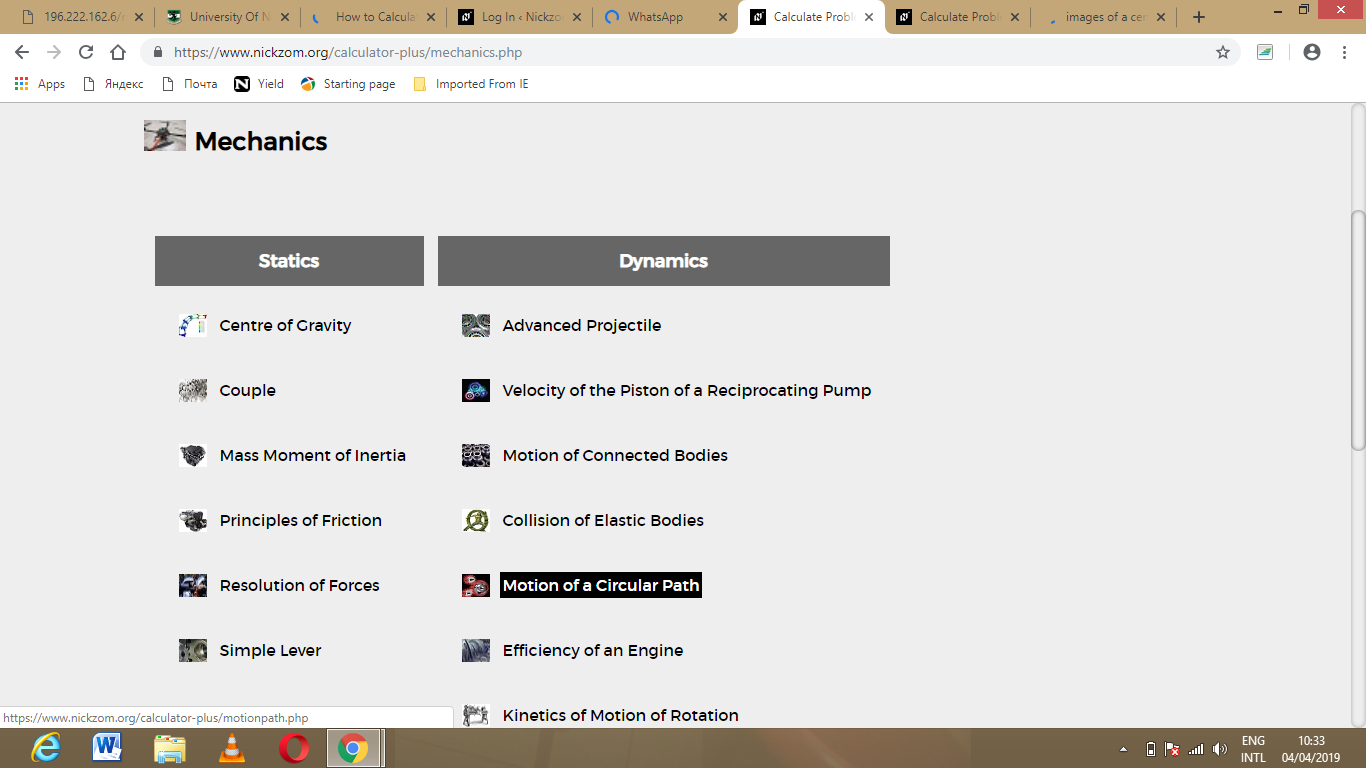Click on Maximum Velocity under Motion of Circular PathThe screenshot below displays the page or activity to enter your value, to get the answer for the maximum velocity according to the respective parameter which are the coefficient of friction (μ), radius (r) and acceleration due to gravity (g).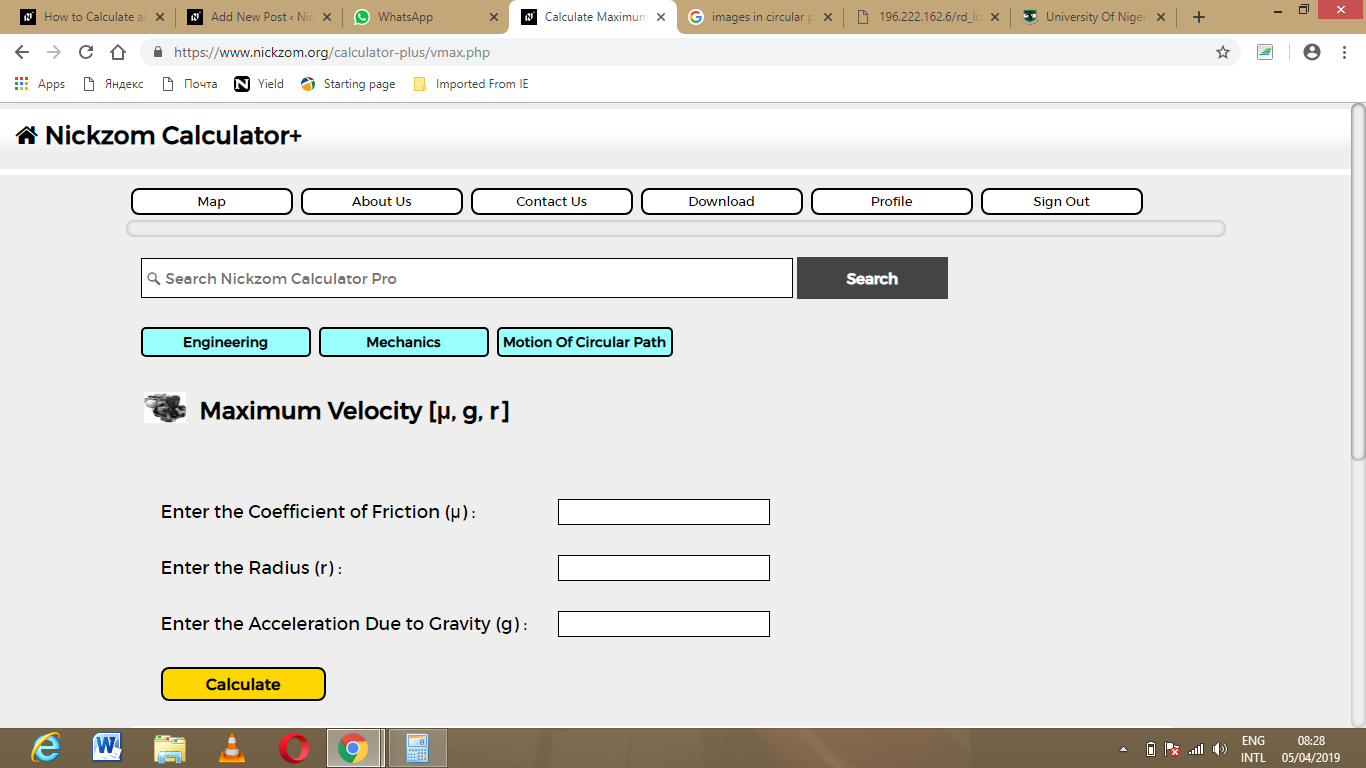Now, enter the value appropriately and accordingly for the parameter as required by the coefficient of friction (μ) is 14, radius (r) is 7 and acceleration due to gravity (g) is 9.8.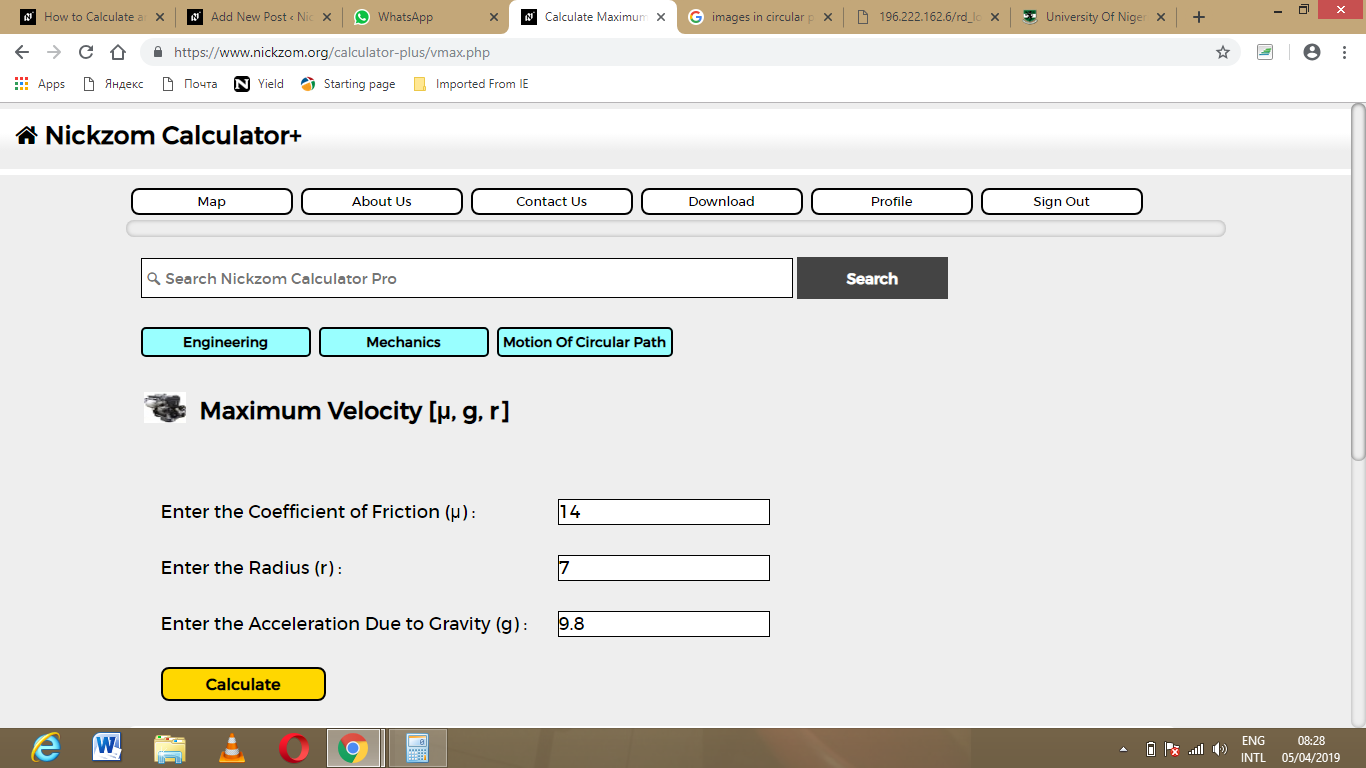Finally, Click on Calculate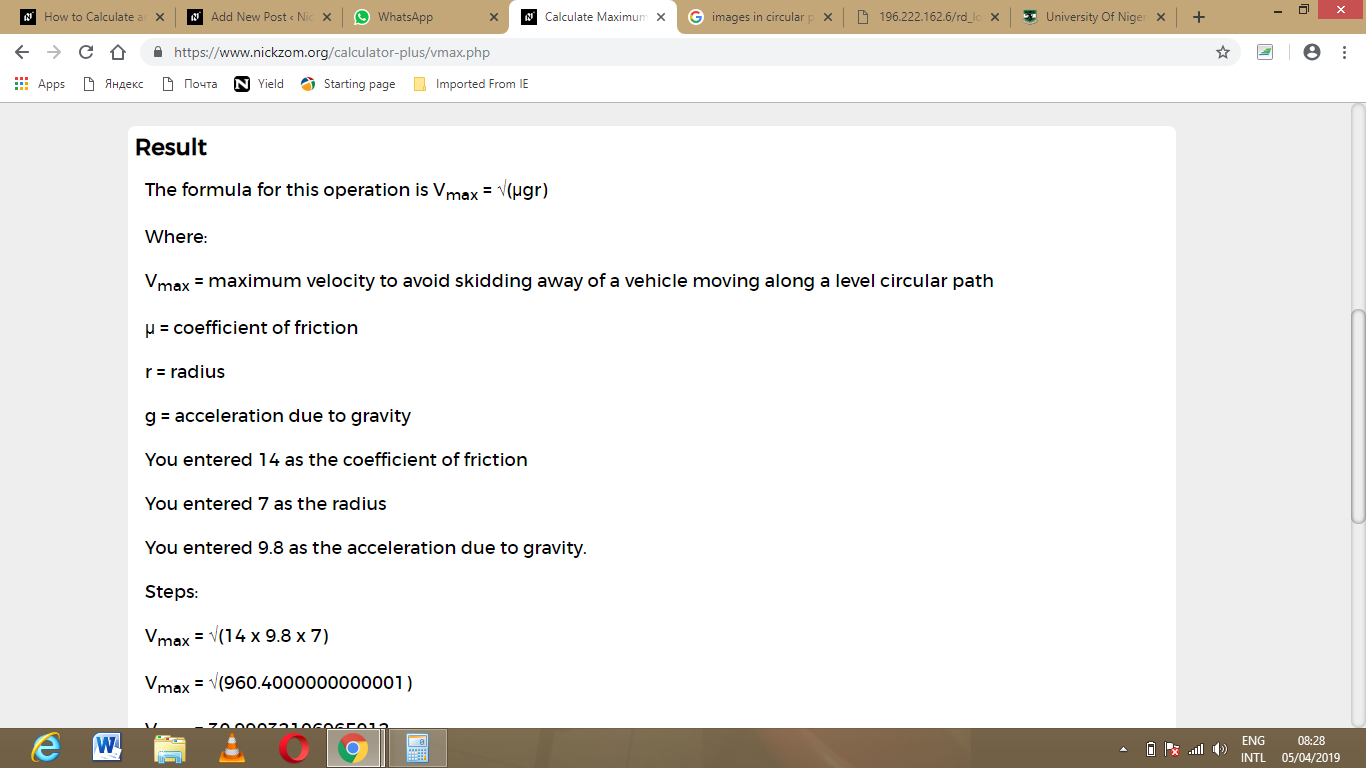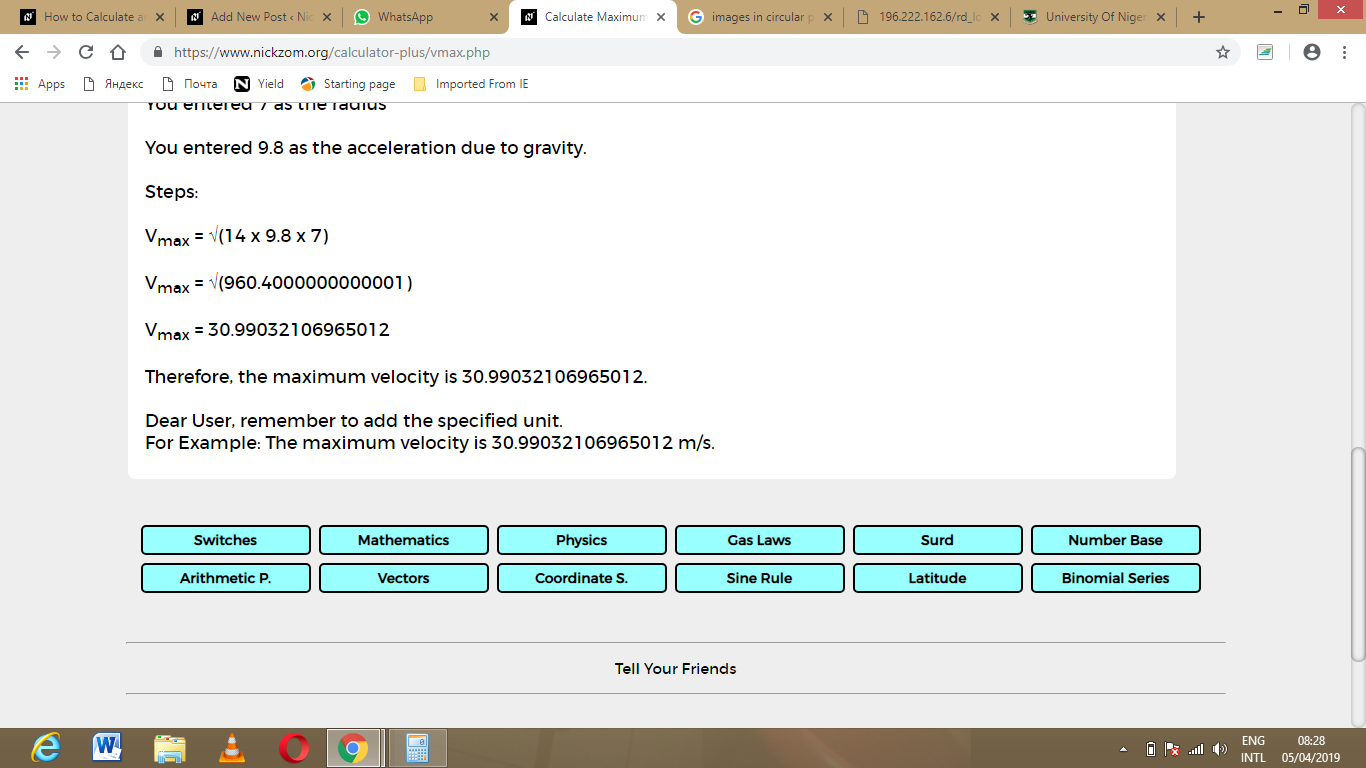As you can see from the screenshot above, Nickzom Calculator – The Calculator Encyclopedia solves for the maximum velocity and presents the formula, workings and steps too.